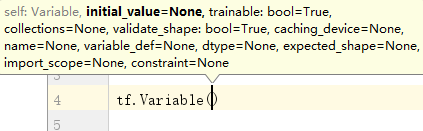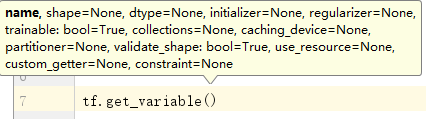• variable共享 tf的Variable是什么就不说了，我们只说下Variable的复用，如下例所示，我们要为图像建立两层卷积网络： def conv_relu(input, kernel_shape, bias_shape): # Create variable named "weights". ...
variable共享
tf的Variable是什么就不说了，我们只说下Variable的复用，如下例所示，我们要为图像建立两层卷积网络：
def conv_relu(input, kernel_shape, bias_shape):
# Create variable named "weights".
weights = tf.Variable(tf.random_normal([5, 5, 32, 32]),
name="conv1_weights")
# Create variable named "biases".
biases = tf.Variable(tf.zeros(), name="conv1_biases")
conv = tf.nn.conv2d(input_images, conv1_weights,
return tf.nn.relu(conv + biases)

def my_image_filter(input_images):
relu1 = conv_relu(input_images, [5, 5, 32, 32], )
relu2 = conv_relu(relu1, [5, 5, 32, 32], )
如果有两个图像需要处理，那么我们调用：
# 创建一套参数
result1 = my_image_filter(image1)
# 在此创建一套参数
result2 = my_image_filter(image2)
我们知道同样作用同样维度的参数我们分别在result1和result2中创建了一次，这样对内存的占用就多了两倍！如何在处理多个图像时仍然使用一套参数呢（也就是variable共享）？？一种选择是建立一套参数的词典，使用唯一的key来确定参数，但这样比较麻烦，并且也不符合封装的思想。有没有一种方法，使得我们可以在代码内部就可以分配给Variable一个类似于key功能的元素？   这时候我们就需要引入get_variable的name属性和variable_scope。
get_variable
我们知道，tf.Variable的name属性是和其对象本身一一对应的。也就是说，如果确定Variable的名字，也就可以确定Variable对象本身了。可能有人会问，那使用tf.Varible(name='xxx')创建Variable对象时，不是给Variable确定的名字了吗？调用相同的创建语句时，岂不是使用相同的名字又创建了一个不同的Variable？看了下面的代码就明白了：
conv1_weights = tf.Variable(tf.random_normal([5, 5, 32, 32]),
name="conv_weights")
print (conv1_weights.name)

conv1_weights = tf.Variable(tf.random_normal([5, 5, 32, 32]),
name="conv_weights")
print (conv1_weights.name)

# 输出：
# conv_weights:0
# conv_weights_1:0
看到没，同样的创建语句，name参数设置也一样，实际的名字属性却不同！也就是说，tf.Variable（）创建方法在会自动在相同name属性下面加序号以方便区别！
如果要使用自己设立一个固定的name属性当如何？
v = tf.get_variable("v", )
print (v.name)
v = tf.get_variable("v", )
print (v.name)
# 输出：v:0
#  ValueError 
可以看出，tf.get_variable()可以实现这样的功能。
variable_scope
我们知道参数的数量常常是巨大的，给参数命名很耗时（给参数起很长的名字并且打出来也很耗时），借助variable_scope我们可以在当前scope下面给所有的variable加上前缀“scope”，这无疑带来了。我们可以看下格式：
with tf.variable_scope("foo"):
v = tf.get_variable("w", )
print (v.name)
# 输出：foo/w:0
reuse参数，tf.get_variable_scope().reuse_variables()以及
回到我们最初的问题--variable共享，怎么在多次调用代码段的同时保持使用同一套Variable呢？？我们有两种方式，一种是设置variable_scope的参数reuse，
1.  reuse==Ture，该scope下强制使用曾经创建过的Variable。一旦发现get_variable的name不是已经创建的或者shape和已经创建的过的variable有不同，则报错。
2. reuse==tf.AUTO_REUSE，如果name已经存在，则复用。如果不存在，则创建。
3. reuse==tf.False，默认为False，该情况下不复用，换句话说，如果name重复了会报错。
另外 tf.get_variable_scope().reuse_variables()和reuse = True的功能是类似的。
参考文献：
 Sharing Variables


展开全文• tensorflow 存储训练数据构建tensorboard中的可视化网络结构 操作变量所属的域变量分享 主要涉及两个函数 * tf.get_variable(name, shape, initializer) ...* tf.variable_scope() ...
tensorflow 存储训练数据和构建tensorboard中的可视化网络结构
操作变量所属的域和变量分享
主要涉及两个函数  * tf.get_variable(name, shape, initializer)
>如果要创建变量的名字存在，则返回已经创建的变量，否则使用参数shape和initializer来创建新的函数

* tf.variable_scope()

管理域名，并且确定变量所在的域。  不同作用域下，变量不共享，所以变量名可以相同。

推荐使用tf.variable_scope来给每一层网络创建一个作用域，并且使用tf.get_variable代替tf.Variable来创建tensorflow变量
例子
不使用上面两个函数： def my_network(input):
W_1 = tf.Variable(tf.random_uniform([784, 100], -1, 1),
name="W_1")
b_1 = tf.Variable(tf.zeros(), name="biases_1")
output_1 = tf.matmul(input, W_1) + b_1
W_2 = tf.Variable(tf.random_uniform([100, 50], -1, 1),
name="W_2")
b_2 = tf.Variable(tf.zeros(), name="biases_2")
output_2 = tf.matmul(output_1, W_2) + b_2
W_3 = tf.Variable(tf.random_uniform([50, 10], -1, 1),
name="W_3")
b_3 = tf.Variable(tf.zeros(), name="biases_3")
output_3 = tf.matmul(output_2, W_3) + b_3
# printing names
print ("Printing names of weight parameters")
print (W_1.name, W_2.name, W_3.name)
print ("Printing names of bias parameters")
print (b_1.name, b_2.name, b_3.name)
return output_3 执行代码输出：     i_1 = tf.placeholder(tf.float32,[1000, 784],name="i_1")
my_network(i_1)
i_2 = tf.placeholder(tf.float32, [1000, 784],name="i_2")
my_network(i_2)
'''
output:
Printing names of weight parameters
W_1:0 W_2:0 W_3:0
Printing names of bias parameters
biases_1:0 biases_2:0 biases_3:0

W_1_1:0 W_2_1:0 W_3_1:0
Printing names of bias parameters
biases_1_1:0 biases_2_1:0 biases_3_1:0
''' 可以看出在第二个网络创建时，将变量名通过添加后缀的形式来创建新的变量。这种tensorflow默认的创建方式会由于变量名的的混乱而会增加可视化工作的难度。使用刚开始两个函数修改此网络     def layer(input, weight_shape, bias_shape):
# 变量初始化器， 将作为get_variable的参数
weight_init = tf.random_uniform_initializer(minval=-1,
maxval=1)
bias_init = tf.constant_initializer(value=0)
# 使用initializer指定上面创建的初始化器
W = tf.get_variable("W", weight_shape,
initializer=weight_init)
b = tf.get_variable("b", bias_shape,
initializer=bias_init)
return tf.matmul(input, W) + b

def my_network(input):
# 为每一层网路创建一个特有的域
with tf.variable_scope("layer_1"):
output_1 = layer(input, [784, 100], )
with tf.variable_scope("layer_2"):
output_2 = layer(output_1, [100, 50], )
with tf.variable_scope("layer_3"):
output_3 = layer(output_2, [50, 10], )
return output_3 将会在最后的代码中看到这种书写风格的巨大优势，注意tensorflow中的域名规则和python中的域名规则相同，一个作用域内可以访问其子作用域中的所有变量，类似于集合的文恩图。 下面看一个例子：  i_1 = tf.placeholder(tf.float32, [1000, 784], name="i_1")
my_network(i_1)

i_2 = tf.placeholder(tf.float32, [1000, 784],name="i_2")
my_network(i_2)
''output
<tensorflow.python.framework.ops.Tensor ...>
ValueError: Over-sharing: Variable layer_1/W already exists...
''' 发现第二个网络输出报错了，因为在创建第二个网络时，作用域的名字和第一个作用域相同，也就是说和第一网络使用同一个作用域，这就导致名字冲突，报错。而这是tensorflow为了安全问题而规定的变量名不可共享。 可以通过函数强制共享：     with tf.variable_scope("shared_variables") as scope:
i_1 = tf.placeholder(tf.float32, [1000, 784], name="i_1")
my_network(i_1)
# 强制共享
scope.reuse_variables()

i_2 = tf.placeholder(tf.float32, [1000, 784], name="i_2")
my_network(i_2) 下面我们介绍存储历史保存数据的tensorflow内置函数，最终是在tensorboard展示的
展开全文python tensorflow
•   tf.Variable()tf.get_variable()都可以用来创建变量，但是前者会自动保证唯一性，而后者不能保证唯一性。 1 tf.VariableVariable(initial_value=None, trainable=True, collections=None, validate_...
  tf.Variable()和tf.get_variable()都可以用来创建变量，但是前者会自动保证唯一性，而后者不能保证唯一性。
1 tf.Variable：
Variable(initial_value=None, trainable=True,
collections=None, validate_shape=True,
caching_device=None,name=None,
expected_shape=None, import_scope=None,
constraint=None)
新建一个变量，变量值是initial_value.
params:
initial_value:初始值

name:可选参数，变量的名字，会自动保证唯一性。
2 tf.get_variable():
get_variable(name, shape=None, dtype=None,
initializer=None, regularizer=None,
trainable=True, collections=None,
caching_device=None, partitioner=None,
validate_shape=True, use_resouce=None,
constraint=None)
获取具有这些参数的现有变量或创建一个新变量。如果该name的变量还未定义，则新创建一个；如果已经定义了，则直接获得该变量。    get_variable()可以用来创建共享变量。
下面用例子来说明二者的不同之处：
import tensorflow as tf

with tf.variable_scope('scope1'):
w1 = tf.Variable(1, name='w1')
w2 = tf.get_variable(name='w2', initializer=2.)

with tf.variable_scope('scope1', reuse=True):
w1_p = tf.Variable(1, name='w1')
w2_p = tf.get_variable(name='w2', initializer=3.)

print('w1', w1)
print('w1_p', w1_p)

print('w2', w2)
print('w2_p', w2_p)

print(w1 is w1_p, w2 is w2_p)
输出结果如下：
w1 <tf.Variable 'scope1/w1:0' shape=() dtype=int32_ref>
w1_p <tf.Variable 'scope1_1/w1:0' shape=() dtype=int32_ref>

w2 <tf.Variable 'scope1/w2:0' shape=() dtype=float32_ref>
w2_p <tf.Variable 'scope1/w2:0' shape=() dtype=float32_ref>

False True
可以看出，tf.Variable()会自动处理冲突问题，如代码中所示，他会将scope1改为scope1_1。
而tf.get_variable()会判断是否已经存在该name的变量，如果有，并且该变量空间的reuse=True,那么就可以直接共享之前的值;如果没有，则重新创建。注意，如果你没有将reuse设为True，则会提示冲突发生。
代码中最后一条语句判断上述变量是否相等，可以看出，通过get_variable()定义的变量是完全等价的，即使后一条get_variable将initializer设为3.，但是由于name=’w2’的变量已经存在，并且reuse=True，则直接引用之前定义的。这样就可以用get_variable()来定义共享变量。
你会发现，tf.get_variable()使用时，一般会和tf.variable_scope()配套使用，需要指定它的作用域空间，这样在引用的使用就可以通过设置指定的scope的reuse=True进行引用。下面讲解一下tf.variable_scope()和tf.name_scope()。
name_scope()是给op_name加前缀，指明op的作用域空间的，op是指操作。而variable_scope()是给get_variable()创建的变量的名字加前缀，表明作用域空间，也可以用于处理命名冲突。
with tf.name_scope('name_test'):
n1 = tf.constant(1, name='cs1')
n2 = tf.Variable(tf.zeros(), name='v1')

nv1 = tf.get_variable(name='nv1', initializer=1.0)

with tf.variable_scope('v_test'):
v_n1 = tf.constant(2, name='cs2')
v_n2 = tf.Variable(tf.zeros(), name='v2')

v1 = tf.get_variable(name='vv1', initializer=2.0)

print('n1', n1.name)
print('n2', n2.name)
print('nv1', nv1.name)

print('v_n1', v_n1.name)
print('v_n2', v_n2.name)
print('v1', v1.name)
输出结果如下：
n1 name_test/cs1:0
n2 name_test/v1:0
nv1 nv1:0

v_n1 v_test/cs2:0
v_n2 v_test/v2:0
v1 v_test/vv1:0
可以看出，variable_scope()不仅对get_variable()有效，对普通的op也有效，能给它们加上前缀，指明作用域空间；而name_scope()仅仅对普通的op有效，对get_variable()无效，不能给后者加上前缀，指明作用空间。
展开全文• tensorflow中有两个关于variable的op，tf.Variable()与tf.get_variable()下面介绍这两个创建变量函数的区别 先来看看这两个函数的参数列表，就不打了，直接截图： 首先有一个区别非常明显： （1） tf.Variable() ...
    tensorflow中有两个关于variable的op，tf.Variable()与tf.get_variable()下面介绍这两个创建变量函数的区别
先来看看这两个函数的参数列表，就不打了，直接截图：首先有一个区别非常明显：
   （1） tf.Variable() 初始化是直接传入initial_value ， 我们使用的时候一般是这样子初始化的：

a = tf.Variable(initial_value=tf.random_normal(shape=[200, 100], stddev=0.1), trainable=True)

（2）tf.get_variable()初始化是传入一个initializer：
    
b = tf.get_variable(name = 'weights', shape=[200, 100], dtype=tf.float32, initializer=tf.random_normal_initializer(stddev=0.1))

当然，明显的区别明显都能看出来，重点是下面这个区别
使用tf.Variable时，如果检测到命名冲突，系统会自己处理。使用tf.get_variable()时，系统不会处理冲突，而会报错
import tensorflow as tf
w_1 = tf.Variable(3,name="w_1")
w_2 = tf.Variable(1,name="w_1")
print w_1.name
print w_2.name
#输出
#w_1:0
#w_1_1:0
import tensorflow as tf

w_1 = tf.get_variable(name="w_1",initializer=1)
w_2 = tf.get_variable(name="w_1",initializer=2)
#错误信息
#ValueError: Variable w_1 already exists, disallowed. Did
#you mean to set reuse=True in VarScope?
所以当我们需要共享变量的时候，需要使用tf.get_variable()。在其他情况下，这两个的用法是一样的。为了方便变量管理，tensorflow 还有一个变量管理器，叫做tf.variable_scope ,举个栗子：

import tensorflow as tf

with tf.variable_scope("scope1"): # scopename is scope1
w1 = tf.get_variable("w1", shape=[])
w2 = tf.Variable(0.0, name="w2")
with tf.variable_scope("scope1", reuse=True):
w1_p = tf.get_variable("w1", shape=[])
w2_p = tf.Variable(1.0, name="w2")

print(w1 is w1_p, w2 is w2_p)  # True False
这就是这两个函数的区别了，在构建网络的时候用起来，你就能够更加深入的了解他们的区别。


展开全文tensorflow
• 一、tf.Variable() （1）参数说明 tf.Variable是一个Variable类。通过变量维持图graph的状态，以便在sess.run()中执行；可以用Variable类创建一个实例在图中增加变量； Args参数说明: initial_value：Tensor或可...tensorflow
• Variabletensorflow中有两个关于variable的op，tf.Variable()与tf.get_variable()下面介绍这两个的区别tf.Variable与tf.get_variable()tf.Variable(initial_value=None, trainable=True, collections=None, validate...
• tf创建变量主要有两种方式:tf.Variable()(V大写)、tf.get_variable()。 1、tf.Variable() （1）每次调用得到的都是不同的变量，即使使用了相同的变量名，在底层实现的时候还是会为变量创建不同的别名var1 = tf....
• 从两个方法的名称上，可以简单理解一下，Variable是定义变量，而get_variable是获取变量（只不过如果获取不到就重新定义一个变量），如果按照这种逻辑，已经基本上可以理解两者的差异了。 下面我们通过一些代码，来...
• tensorflow中有很多需要变量共享的场合，比如在多个GPU上训练网络时网络参数... tf.get_variable函数的作用从tf的注释里就可以看出来-- ‘Gets an existing variable with this name or create a new one’。 与...
• tf.get_variable用来创建或者获取变量 #定义的基本等价 v = tf.get_variable("v",shape=,initializer.constant_initializer(1.0)) v = tf.Variable(tf.constant(1.0,shape=),name="v"...
• 基于源码修改JDBC8驱动连接Mycat1.6报错 Unknown system variable 'query_cache_size' ，配置好mycat相应配置直接bin目录启动即可
• tensorflow中有两个关于variable的op，tf.Variable()与tf.get_variable()下面介绍这两个的区别 使用tf.Variable时，如果检测到命名冲突，系统会自己处理。使用tf.get_variable()时，系统不会处理冲突，而会报错 ...TensorFlow
• 一 实例分别使用Variable定义变量使用get_variable来定义变量二 代码import tensorflow as tf tf.reset_default_graph() var1 = tf.Variable(1.0 , name='firstvar') print ("var1:",var1.name) var1 = ...
• 文章目录Variable与get_variablename_scope与variable_scope Variable与get_variable 直接上代码： #!/usr/bin/env python # -*- coding: utf-8 -*- """ @Time : 2018/11/13 9:18 @Author : Li ...tensorflow
• 2、tf.variable_scope() 3、tf.variable_scope()函数嵌套 1、tf.get_variable() tf.get_variable()用来创建变量时，tf.Variable()函数的功能基本等价。 v = tf.get_variable("v", shape=, initializer=tf....
• tensorflow中有两个关于variable的op，tf.Variable()与tf.get_variable()下面介绍这两个的区别 tf.Variable与tf.get_variable() tf.Variable(initial_value=None, trainable=True, collections=None, validate_...
• 1. tf.Variable()W = tf.Variable(, name=)用于生成一个初始值为initial-value的变量。必须指定初始化值2.tf.get_variable() W = tf.get_variable(name, shape=None, dtype=tf.float32, initializer=None,tensorflow 共享变量 reuse
• Variable 最近在学习TensorFlow的过程中， 看到在...tf.get_variable()tf.Variable()。 def weight_variable(shape): #initial = tf.truncated_normal(shape, stddev=0.1) #return tf.Variable(initial) ...tensorflow
• 共享变量 你可以在怎么使用变量中所描述的...本教程就是演示如何使用tf.variable_scope() tf.get_variable()两个方法来实现这一点. 问题 假设你为图片过滤器创建了一个简单的模块，我们的卷积神经网络
• tensorflow中有两个关于variable的op，tf.Variable()与tf.get_variable()下面介绍这两个的用法与区别： tf.Variable() W = tf.Variable(&amp;lt;initial-value&amp;gt;, name=&amp;lt;...tensorflow
• 最近在学习使用TensorFlow，一直没有搞明白其中的get_variable()与Variable()的区别，今天特意在网上搜了一些资料，这里将一篇写的简单易懂的一篇外国博客分享给大家：...为了巩固自己的理解，自己...python
• 上上篇博文也写了这个话题，这次自己又敲了一下代码，再次研究了一下关于tf.Variable()  tf.get_variable...with tf.variable_scope('aa') as scope: w = tf.get_variable("a1", [1, 2]) va...TensorFlow
• tensorflow中的变量 ...2 tf.variable_scope()与tf.get_variable的配合使用 3 使用tf.get_variable的好处 tensorflow中的变量 1 tf.Variable与tf.get_variable创建变量 tf.Variable...
• 转自 ... Variable tensorflow中有两个关于variable的op，tf.Variable()与tf.get_variable()下面介绍这两个的区别 tf.Variable与tf.get_variable() tf.Variable(initial_v
•tensorflow 创建变量
• get_variable() tf.get_variable(name, shape, initializer): name就是变量的名称，shape是变量的维度，initializer是变量初始化的方式，初始化的方式有以下几种： tf.constant_initializer：常量初始化函数 tf....
• ## tf.get_variable()函数

千次阅读 2019-07-29 15:47:44
可使用tf.get_variable() 函数代替tf.Variable()。如果变量存在，函数tf.get_variable( ) 会返回现有的变量。如果变量不存在，会根据给定形状初始值创建变量。 tf.get_variable( name, shape=None, dtype=......